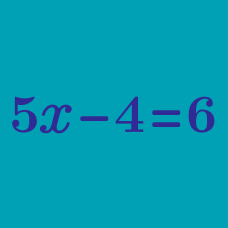Algebra

# Isolating a Variable

Solve the following for $$Y$$ in terms of $$X:$$ $X = 6Y .$

Given the equation $$X = 2 Y + 7,$$ solve for $$Y$$ in terms of $$X$$.

Solve the following for $$Y$$ in terms of $$X:$$ $\frac{Y+2}{X+7} = 1.$

Joshua has 5 times as many toys as Kevin.

Joshua has 8 more toys than Larry.

If Larry has $$L$$ toys and Kevin has $$K$$ toys, which of the following expresses $$K$$ in terms of $$L$$?

Given the equation $$X = 3 Y + 6,$$ solve for $$Y$$ in terms of $$X$$.

×